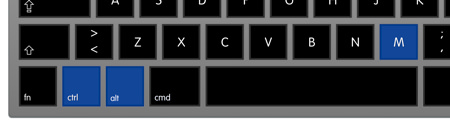# Equation to LaTeX

Extract equations from PDFs or handwritten notes in seconds just by taking a screenshot.

Paste the resulting LaTeX code into any Markdown or LaTeX editor.

• “If I had known about Mathpix earlier, perhaps I would have had enough time to work out the Grand Unified Theory.”

#### Albert Einstein

• “When I lost my .tex file to the Principia, I was devastated. Mathpix helped me effortlessly use equations from the Principia in my new work. I now have more time to stand beneath trees and get hit by apples.”

#### Isaac Newton

• “Mathpix's AI definitely passes this Turing test!”

## Go from image to LaTeX in a few simple steps...

Digitize any math or text on your screen in seconds.

• 1

## Start

by clicking the screenshot button

You can find it in the top left cornerOr use the keyboard shortcut:

Ctrl + ⌘ + M on Mac

Ctrl + Alt + M on Windows & Linux• 2

## Capture

your desired inputs within the screenshot box

Just click and drag

• 3

## Find

The new list view allows you to navigate all your Snips quickly and easily.

• 4

## Paste

the LaTeX into any compatible editor

Once you've chosen your desired format, just paste! The LaTeX or text is already copied to your clipboard.

## Mobile Snip digitizes your handwriting, syncs with web and desktop

Perfect for including text and equations from notebooks or whiteboards in your typed notes.

## Drag images directly into the web editor to digitize

You can also paste results into your documents by clicking to insert them from the Snip sidebar.

## Recognition features

Snip can very accurately convert images of simple and complicated printed math, text, and tables.

It can also convert images of simple handwritten math, text, and tables.

\Gamma _ { \epsilon } ( x ) = [ 1- e ^ { - 2\pi \epsilon } ] ^ { 1- x } \prod _ { n = 0} ^ { \infty } \frac { 1- \operatorname{exp} ( - 2\pi \epsilon ( n + 1) ) } { 1- \operatorname{exp} ( - 2\pi \epsilon ( x + n ) ) }

\left( \begin{array} c t ^ { \prime } \\ x ^ { \prime } \\ y ^ { \prime } \\ z ^ { \prime } \end{array} \right) = \left( \begin{array} { c c c c } { \gamma } & { - \gamma \beta } & { 0 } & { 0 } \\ { - \gamma \beta } & { \gamma } & { 0 } & { 0 } \\ { 0 } & { 0 } & { 1 } & { 0 } \\ { 0 } & { 0 } & { 0 } & { 1 } \end{array} \right) \left( \begin{array} c t \\ x \\ y \\ z \end{array} \right)

\ell ( \theta ) = \sum _ { i = 1} ^ { m } \operatorname{log} p ( x ; \theta )

6 \mathrm { CO } _ { 2 } + 6 \mathrm { H } _ { 2 } \mathrm { O } \rightarrow \mathrm { C } _ { 6 } \mathrm { H } _ { 12 } \mathrm { O } _ { 6 } + 6 \mathrm { O } _ { 2 }

We can also read large chunks of text that contain multiple lines. This is really helpful for when you want to extract a chunk of text from a PDF because you are unable to copy the source text directly.

% We even support basic tables!
\begin{tabular}{l|l}
Hyperparameter & Value \\
\hline Horizon (T) & 2048 \\
Adam stepsize & $$3 \times 10^{-4}$$ \\
Num. epochs & 10 \\
Minibatch size & 64 \\
Discount $$(\gamma)$$ & 0.99 \\
GAE parameter $$(\lambda)$$ & 0.95
\end{tabular}

You can find more examples of images that Snip can digitize accurately in the User Guide.

## App Features

Snip supports more output formats than just text and LaTeX

• ## Input formats:

- Image of printed math

- Image of printed text (Latin alphabet languages)

- Image of mixed printed text and math

- Image of printed tables

- Image of simple handwritten math

- Image of simple handwritten text

- Image of simple handwritten tables

- LaTeX

- Markdown

- Simple math, text, tables drawn in app

• ## Output formats:

- LaTeX

- Text

- Markdown

- MathML

- Asciimath

- SVG

- HTML

- TSV

- PNG

- Image URL

- Markdown Image URL

- HTML Image URL

## Use Snip with any LaTeX or Markdown editor

• Overleaf

• Microsoft Word

• Typora

• TeXmaker

• StackEdit

• MacDown

• Authorea

• TeXstudio

• TeXworks

and any other editors that accept LaTeX, MathML, or Asciimath.

## Individual and Organizational plans

Choose the plan that’s right for you!

• ## Free

Best plan for most users

• 100 snips
• ## Pro

Great for STEM professionals!

#### per month

• First 2 users included
3-49 Users: $4.49 / user 50+ Users:$3.99 / user
Centralized user management
Cancel anytime
##### GET IT NOW

• “I tested AWS Textract, Azure Computer Vision, and Google Cloud Vision and Mathpix snip actually came out way ahead.”

#### Nathan Graham

##### Director at Whiting School of Engineering, Johns Hopkins
• “This is changing my life. Mathpix's "Snip" will let you screenshot an equation, and it will return the LaTeX code. Works passably on handwriting, and nearly flawlessly on pdf equations.”

#### Sean Carroll

##### Physicist, Caltech Professor & Author of
Something Deeply Hidden
• “A new product Mathpix, seems to be more accurate than InftyReader!”